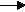gcsescience.com                                       8                                       gcsescience.com

Rates of Reaction

What is the Effect of Increasing the Concentration
on the Rate of a Chemical Reaction?

If the concentration of a substance is increased then
there will be more particles of the substance per dm3 in
the solution. The more particles that there are in the
same volume, then the closer to each other the particles
will be. This means that the particles will collide
more frequently and the rate of the reaction increases.

The effect of increasing the concentration on the rate of
a reaction can be shown by looking at the reaction between
sodium thiosulfate solution and dilute hydrochloric acid.
The balanced equation for this reaction is shown below.

HCl + sodium thiosulfatesodium chloride + sulfur dioxide + sulfur + water.
2HCl(aq)  +  Na2S2O3(aq)2NaCl(aq)    +      SO2(g)    +   S(s)  +  H2O(l)

Solid sulfur (S(s)) is formed in the flask as one of the products.
Increasing the concentration of sodium thiosulfate means
that the solid sulfur will be produced more quickly and there
will be less time before the cross can no longer be seen.When making different concentrations of sodium thiosulfate
solution for this experiment, a fair test must use the
same total volume of sodium thiosulfate plus hydrochloric acid.

gcsescience.com      The Periodic Table      Index      Reaction Rate Quiz      gcsescience.com